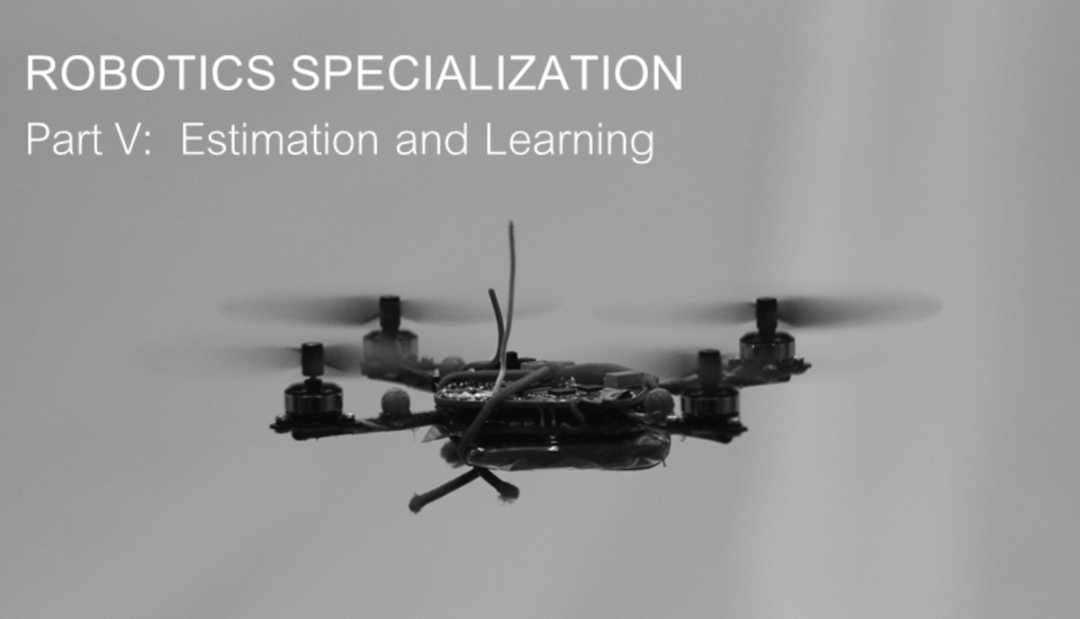Coursera上的课程（Robotics: Estimation and Learning），感觉讲得特别棒，写下自己的理解。

• 介绍如何使用一元高斯分布、多元高斯分布和高斯混合模型对机器人误差进行建模。
• 介绍求解这些高斯分布的算法。
• 以小球检测这一实际应用来实践我们的模型和算法。

1. 一元高斯分布

• 高斯分布使得只需要两个参数就能确定一个连续的概率分布。这两个参数分别是均值和方差，这很简洁。这也使得高斯分布易于计算和推断。
• 高斯分布有一些较好的数学性质。例如，两个高斯分布的积还是高斯分布。这使得你在对高斯分布做运算的时候不需要考虑其他的概率分布。
• 理论上来说，根据中心极限定理，大量相互独立的随机变量，其均值的分布以高斯分布为极限。而噪声和不确定性就是包含大量相互独立的随机变量的数据，用高斯分布对它们进行建模是不二选择。## 2. 求解一元高斯分布：最大似然估计带入函数得到函数，其取最小值时关于的导数等于0：## 3. 多元高斯分布## 4. 求解多元高斯分布：最大似然估计## 5. 高斯混合模型## 6. 求解高斯混合模型：EM算法## 7. 实战：小球检测

（注：我们使用matlab完成所有代码）

imagepath = './train';
Samples = [];
for k=1:19
R = I(:,:,1);
G = I(:,:,2);
B = I(:,:,3);

% Collect samples
disp('');
disp('INTRUCTION: Click along the boundary of the ball. Double-click when you get back to the initial point.')
disp('INTRUCTION: You can maximize the window size of the figure for precise clicks.')

sample_ind = find(mask > 0); % select marked pixels
R = R(sample_ind);
G = G(sample_ind);
B = B(sample_ind);

Samples = [Samples; [R G B]]; % insert selected pixels into samples

disp('INTRUCTION: Press any key to continue. (Ctrl+c to exit)')
pause
end

save('Samples.mat', 'Samples'); % save the samples to file

figure,
scatter3(Samples(:,1),Samples(:,2),Samples(:,3),'.');
title('Pixel Color Distribubtion');
xlabel('Red');
ylabel('Green');
zlabel('Blue');


mu = mean(Samples); % mu

sig=zeros(3,3); % sigma
for i=1:N
data=double(Samples(i,:));
sig=sig+(data-mu)'*(data-mu)/N;
end

% save the coefficients to files
save('mu.mat', 'mu');
save('sig.mat', 'sig');

function [segI, loc] = detectBall(I)

Id=double(I); % array in size of (row, col, 3)

row=size(Id,1);
col=size(Id,2);

% x_i - mu
for i=1:3
Id(:,:,i) = Id(:,:,i) - mu(i);
end

% reshape the image to a matrix in size of (row*col, 3)
Id=reshape(Id,row*col,3);

% calc possibility using gaussian distribution
% be careful of using * and .* in matrix multiply
Id = exp(-0.5* sum(Id*inv(sig).*Id, 2)) ./ (2*pi)^1.5 ./ det(sig)^0.5;

% reshape back, now each pixels is with the value of the possibility
Id=reshape(Id,row,col);

% set threshold
thr=8e-06;

% binary image about if each pixel 'is ball'
Id=Id>thr;

% find the biggest ball area
segI = false(size(Id));

CC = bwconncomp(Id);
numPixels = cellfun(@numel,CC.PixelIdxList);
[biggest,idx] = max(numPixels);
segI(CC.PixelIdxList{idx}) = true;
%figure, imshow(segI); hold on;

S = regionprops(CC,'Centroid');
loc = S(idx).Centroid;
%plot(loc(1), loc(2),'r+');

imagepath = './train';
for k=1:19
[segI, loc] = detectBall(I);
figure, imshow(segI); hold on;
plot(loc(1), loc(2), '+b','MarkerSize',7);
disp('Press any key to continue. (Ctrl+c to exit)')
pause
end